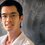Can a triangle be formed by the three special cevians of a triangle, altitude,median,angle bisector...?Note by Kishan K
6 years, 3 months ago

This discussion board is a place to discuss our Daily Challenges and the math and science related to those challenges. Explanations are more than just a solution — they should explain the steps and thinking strategies that you used to obtain the solution. Comments should further the discussion of math and science.

When posting on Brilliant:

• Use the emojis to react to an explanation, whether you're congratulating a job well done , or just really confused .
• Ask specific questions about the challenge or the steps in somebody's explanation. Well-posed questions can add a lot to the discussion, but posting "I don't understand!" doesn't help anyone.
• Try to contribute something new to the discussion, whether it is an extension, generalization or other idea related to the challenge.
• Stay on topic — we're all here to learn more about math and science, not to hear about your favorite get-rich-quick scheme or current world events.

MarkdownAppears as
*italics* or _italics_ italics
**bold** or __bold__ bold

- bulleted
- list

• bulleted
• list

1. numbered
2. list

1. numbered
2. list
Note: you must add a full line of space before and after lists for them to show up correctly
paragraph 1

paragraph 2

paragraph 1

paragraph 2

> This is a quote
This is a quote
# I indented these lines
# 4 spaces, and now they show
# up as a code block.

print "hello world"
# I indented these lines
# 4 spaces, and now they show
# up as a code block.

print "hello world"
MathAppears as
Remember to wrap math in $$...$$ or $...$ to ensure proper formatting.
2 \times 3 $2 \times 3$
2^{34} $2^{34}$
a_{i-1} $a_{i-1}$
\frac{2}{3} $\frac{2}{3}$
\sqrt{2} $\sqrt{2}$
\sum_{i=1}^3 $\sum_{i=1}^3$
\sin \theta $\sin \theta$
\boxed{123} $\boxed{123}$

Sort by:

Yes, it can be drawn!!!

- 6 years, 3 months ago

I suppose you mean the altitude, median and bisector of just one angle? As opposed to one cevian from each angle.

- 6 years, 3 months ago

what is median

- 6 years, 3 months ago

A median of a triangle is a line segment joining a vertex to the midpoint of the opposing side.

- 6 years, 3 months ago

See this video.

- 6 years, 3 months ago

I'm kinda surprised that you know what is cevian and not median. ;O

- 6 years, 3 months ago

Yes indeed. You can get expressions of the lengths of sides if the 3 medians or bisectors are given. Solve them. Then you know the length of each side.

- 6 years, 3 months ago

i think in Equilateral triangle median is angle bisector and altitude

- 6 years, 3 months ago

yes, in equilateral triangle it is possible i think.As median itself makes angle bisector and also the altitude. Am i right?

- 6 years, 3 months ago

Is your question asking if three of the same type of cevian (e.g. 3 medians) form a triangle, i.e. be non-concurrent, or if three of any type of cevian? If the latter then yes. I'm not sure about the former yet.

- 6 years, 3 months ago

I think he is trying to ask that if we draw an altitude from A , a median from B ,and an angle bisector of C.Then will they form a triangle.?Correct me if i am wrong.Obviously it will not form in equilateral triangle.

- 6 years, 3 months ago

Well yes it would be possible in both cases. If we knew the lengths and the angles. even if it was equilateral. simple Sine and Cosine rules can be used to determine those lines that thus make the triangle.

- 6 years, 3 months ago

Simple Sin or Cosin rule can be used to determine those lengths.

- 6 years, 3 months ago

it can be drawn

- 6 years, 3 months ago

yes

- 5 years, 5 months ago